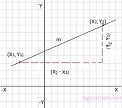# Find Slope of A Straight Line

Calculator to find the slope of the straight line using the values of x and y coordinates.
 Y2: Y1: X2: X1:

 Line Slope (m) :

The important properties of a straight line is in how it angles away from the horizontal. This concept is reflected in something called the "slope" of the line.

We can find the slop (m) using formula :

$$m = \frac{(y_2 - y_1)}{(x_2 - x_1)} or \frac{(y_1 - y_2)}{(x_1 - x_2)}$$Let's take the example to find out the slop of line passing through two ponits coordinates are (-4, 3) and (9, 8):
$$m = \frac{(y_2 - y_1)}{(x_2 - x_1)}$$ $$m = \frac{(8 - 3)}{(9 - (-4))}$$ $$m = \frac{(5)}{(9 + 4)}$$ $$m = \frac{5}{13}$$ $$m = 0.3846153846153846$$ $$m = 0.385$$
$$\text{or m }= \frac{(y_1 - y_2)}{(x_1 - x_2)}$$ $$m = \frac{(3 - 8)}{((-4) - 9)}$$ $$m = \frac{(-5)}{(-13)}$$ $$m = \frac{5}{13}$$ $$m = 0.3846153846153846$$ $$m = 0.385$$